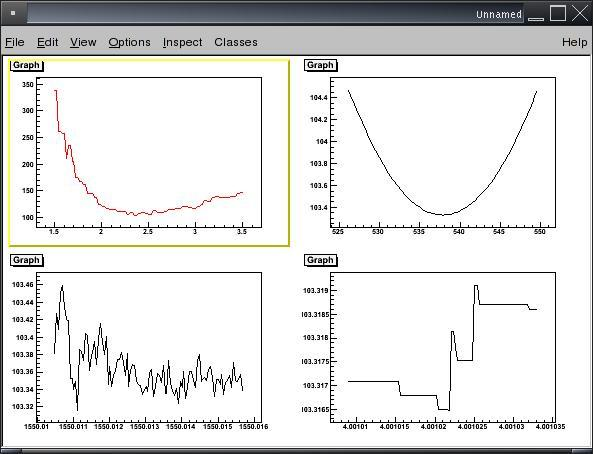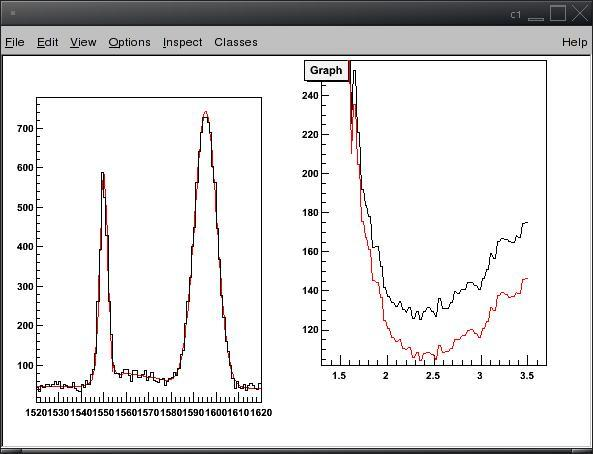# Fitting does not converge due to non-smoth fitting function

Hi,

I trying to fit a function that is a gaussian convoluted with a slice of a two D histogram. Which slice is a parameter of the function, as is the width of the gaussian etc. The function also has a linear background and an arbitrary number of additional gaussians. My function represents the data in a nice why (see function1.jpg) but if one takes alook at a scan of the four parameters that determines the shape of the peak to the left (see parameterscans.jpg, counting from upper left clockwise, “slice”, amplitude, position, and width) it is obvious that minuit will have troubles finding the minimum. Is there a “standard” way around this?

cheers

Joaand in particular the way errors are defined.

Rene

Hi again,

I see the problem as slightly more generic than my code. I have a function that is a convolution between a gaussian and a histogram. Such a function will often not be mathematically strictly continious in the first derivative with respect to its parameters, and this causes problems since the chi2 no longer will be continious with respect to these derivatives… and this gives me my wiggles in the scan of chi2 vs the parameters. So the question really was, is there a standard why of dealing with this using minuit? I have tried to implement a chi2 function that fits a second degree polynom to the chi2 values at a few points around the present parameter value, and use this polynom to calculate the chi2 that minuit is trying to find a minimum for, but without sucess. Any other, better, ideas?

cheers

Joa

Hi,

the MIGRAD algorithm of Minuit requires the derivatives to be continous and you will have a problem in that case. You can try eventually the Simplex algorithm which does not require the derivatives.

However, I would smooth your histogram , or better directly the data going in the histogram to have then a chi2 function with continous derivatives.

Best Regards,

Lorenzo